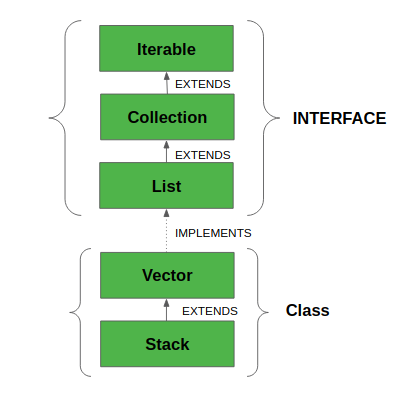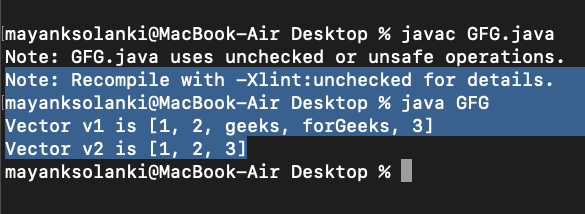Open In App

# Vector Class in Java

The Vector class implements a growable array of objects. Vectors fall in legacy classes, but now it is fully compatible with collections. It is found in java.util package and implement the List interface, so we can use all the methods of the List interface as shown below as follows:• Vector implements a dynamic array which means it can grow or shrink as required. Like an array, it contains components that can be accessed using an integer index.
• They are very similar to ArrayList, but Vector is synchronized and has some legacy methods that the collection framework does not contain.
• It also maintains an insertion order like an ArrayList. Still, it is rarely used in a non-thread environment as it is synchronized, and due to this, it gives a poor performance in adding, searching, deleting, and updating its elements.
• The Iterators returned by the Vector class are fail-fast. In the case of concurrent modification, it fails and throws the ConcurrentModificationException.

Syntax:

`public class Vector<E> extends AbstractList<E> implements List<E>, RandomAccess, Cloneable, Serializable`

Here, E is the type of element.

• It extends AbstractList and implements List interfaces.
• It implements Serializable, Cloneable, Iterable<E>, Collection<E>, List<E>, RandomAccess interfaces.
• The directly known subclass is Stack.

Important points regarding the Increment of vector capacity are as follows:

If the increment is specified, Vector will expand according to it in each allocation cycle. Still, if the increment is not specified, then the vector’s capacity gets doubled in each allocation cycle. Vector defines three protected data members:

• int capacityIncreament: Contains the increment value.
• int elementCount: Number of elements currently in vector stored in it.
• Object elementData[]: Array that holds the vector is stored in it.

Common Errors in the declaration of Vectors are as follows:

• Vector throws an IllegalArgumentException if the InitialSize of the vector defined is negative.
• If the specified collection is null, It throws NullPointerException.

### Constructors

1. Vector(): Creates a default vector of the initial capacity is 10.

`Vector<E> v = new Vector<E>();`

2. Vector(int size): Creates a vector whose initial capacity is specified by size.

`Vector<E> v = new Vector<E>(int size);`

3. Vector(int size, int incr): Creates a vector whose initial capacity is specified by size and increment is specified by incr. It specifies the number of elements to allocate each time a vector is resized upward.

`Vector<E> v = new Vector<E>(int size, int incr);`

4. Vector(Collection c): Creates a vector that contains the elements of collection c.

vect

`Vector<E> v = new Vector<E>(Collection c);`

### Methods in Vector Class

Let us first discuss and implement how to create and use a Vector prior to landing upon the methods of this class.

Example:

## Java

 `// Java Program to Demonstrate Working of Vector``// Via Creating and Using It` `// Importing required classes``import` `java.io.*;``import` `java.util.*;` `// Main class``class` `GFG {` `    ``// Main driver method``    ``public` `static` `void` `main(String[] args)``    ``{``        ``// Size of the Vector``        ``int` `n = ``5``;` `        ``// Declaring the Vector with``        ``// initial size n``        ``Vector v = ``new` `Vector(n);` `        ``// Appending new elements at``        ``// the end of the vector``        ``for` `(``int` `i = ``1``; i <= n; i++)``            ``v.add(i);` `        ``// Printing elements``        ``System.out.println(v);` `        ``// Remove element at index 3``        ``v.remove(``3``);` `        ``// Displaying the vector``        ``// after deletion``        ``System.out.println(v);` `        ``// iterating over vector elements``        ``// using for loop``        ``for` `(``int` `i = ``0``; i < v.size(); i++)` `            ``// Printing elements one by one``            ``System.out.print(v.get(i) + ``" "``);``    ``}``}`

Output

```[1, 2, 3, 4, 5]
[1, 2, 3, 5]
1 2 3 5```

Note:

• If the vector increment is not specified then it’s capacity will be doubled in every increment cycle.
• The capacity of a vector cannot be below the size, it may equal to it.

### Performing Various Operations on Vector class in Java

Let us discuss various operations on Vector class that are listed as follows:

2. Updating elements
3. Removing elements
4. Iterating over elements

In order to add the elements to the Vector, we use the add() method. This method is overloaded to perform multiple operations based on different parameters. They are listed below as follows:

• add(Object): This method is used to add an element at the end of the Vector.
• add(int index, Object): This method is used to add an element at a specific index in the Vector.

Example:

## Java

 `// Java Program to Add Elements in Vector Class` `// Importing required classes``import` `java.io.*;``import` `java.util.*;` `// Main class``// AddElementsToVector``class` `GFG {` `    ``// Main driver method``    ``public` `static` `void` `main(String[] arg)``    ``{` `        ``// Case 1``        ``// Creating a default vector``        ``Vector v1 = ``new` `Vector();` `        ``// Adding custom elements``        ``// using add() method``        ``v1.add(``1``);``        ``v1.add(``2``);``        ``v1.add(``"geeks"``);``        ``v1.add(``"forGeeks"``);``        ``v1.add(``3``);` `        ``// Printing the vector elements to the console``        ``System.out.println(``"Vector v1 is "` `+ v1);` `        ``// Case 2``        ``// Creating generic vector``        ``Vector v2 = ``new` `Vector();` `        ``// Adding custom elements``        ``// using add() method``        ``v2.add(``1``);``        ``v2.add(``2``);``        ``v2.add(``3``);` `        ``// Printing the vector elements to the console``        ``System.out.println(``"Vector v2 is "` `+ v2);``    ``}``}`

Output:```Vector v1 is [1, 2, geeks, forGeeks, 3]
Vector v2 is [1, 2, 3]```

Operation 2: Updating Elements

After adding the elements, if we wish to change the element, it can be done using the set() method. Since a Vector is indexed, the element which we wish to change is referenced by the index of the element. Therefore, this method takes an index and the updated element to be inserted at that index.

Example

## Java

 `// Java code to change the``// elements in vector class` `import` `java.util.*;` `public` `class` `UpdatingVector {``  ` `    ``public` `static` `void` `main(String args[])``    ``{``        ``// Creating an empty Vector``        ``Vector vec_tor = ``new` `Vector();` `        ``// Use add() method to add elements in the vector``        ``vec_tor.add(``12``);``        ``vec_tor.add(``23``);``        ``vec_tor.add(``22``);``        ``vec_tor.add(``10``);``        ``vec_tor.add(``20``);` `        ``// Displaying the Vector``        ``System.out.println(``"Vector: "` `+ vec_tor);` `        ``// Using set() method to replace 12 with 21``        ``System.out.println(``"The Object that is replaced is: "``                        ``+ vec_tor.set(``0``, ``21``));` `        ``// Using set() method to replace 20 with 50``        ``System.out.println(``"The Object that is replaced is: "``                        ``+ vec_tor.set(``4``, ``50``));` `        ``// Displaying the modified vector``        ``System.out.println(``"The new Vector is:"` `+ vec_tor);``    ``}``}`

Output

```Vector: [12, 23, 22, 10, 20]
The Object that is replaced is: 12
The Object that is replaced is: 20
The new Vector is:[21, 23, 22, 10, 50]```

Operation 3: Removing Elements

In order to remove an element from a Vector, we can use the remove() method. This method is overloaded to perform multiple operations based on different parameters. They are:

• remove(Object): This method is used to remove an object from the Vector. If there are multiple such objects, then the first occurrence of the object is removed.
• remove(int index): Since a Vector is indexed, this method takes an integer value which simply removes the element present at that specific index in the Vector. After removing the element, all the elements are moved to the left to fill the space and the indices of the objects are updated.

Example

## Java

 `// Java code illustrating the removal``// of elements from vector` `import` `java.util.*;``import` `java.io.*;` `class` `RemovingElementsFromVector {``  ` `    ``public` `static` `void` `main(String[] arg)``    ``{` `        ``// create default vector of capacity 10``        ``Vector v = ``new` `Vector();` `          ``// Add elements using add() method``        ``v.add(``1``);``        ``v.add(``2``);``        ``v.add(``"Geeks"``);``        ``v.add(``"forGeeks"``);``        ``v.add(``4``);` `        ``// removing first occurrence element at 1``        ``v.remove(``1``);` `        ``// checking vector``        ``System.out.println(``"after removal: "` `+ v);``    ``}``}`

Output:

`after removal: [1, Geeks, forGeeks, 4]`

Operation 4: Iterating the Vector

There are multiple ways to iterate through the Vector. The most famous ways are by using the basic for loop in combination with a get() method to get the element at a specific index and the advanced for a loop.

Example

## Java

 `// Java program to iterate the elements``// in a Vector` `import` `java.util.*;` `public` `class` `IteratingVector {` `    ``public` `static` `void` `main(String args[])``    ``{``          ``// create an instance of vector``        ``Vector v = ``new` `Vector<>();` `          ``// Add elements using add() method``        ``v.add(``"Geeks"``);``        ``v.add(``"Geeks"``);``        ``v.add(``1``, ``"For"``);` `        ``// Using the Get method and the``        ``// for loop``        ``for` `(``int` `i = ``0``; i < v.size(); i++) {` `            ``System.out.print(v.get(i) + ``" "``);``        ``}` `        ``System.out.println();` `        ``// Using the for each loop``        ``for` `(String str : v)``            ``System.out.print(str + ``" "``);``    ``}``}`

Output

```Geeks For Geeks
Geeks For Geeks```

Note: Do give a read to the ArrayList vs Vector class in Java to grasp it better.

In Java, the Vector class is a part of the Java Collections Framework and provides a dynamic array implementation of the List interface. It was added in the original release of Java (Java 1.0) and provides a number of methods for manipulating the elements of a vector, including adding, inserting, and removing elements.

## Java

 `import` `java.util.Vector;` `public` `class` `VectorExample {``    ``public` `static` `void` `main(String[] args) {``        ``// Create a new vector``        ``Vector v = ``new` `Vector<>(``3``, ``2``);` `        ``// Add elements to the vector``        ``v.addElement(``1``);``        ``v.addElement(``2``);``        ``v.addElement(``3``);` `        ``// Insert an element at index 1``        ``v.insertElementAt(``0``, ``1``);` `        ``// Remove the element at index 2``        ``v.removeElementAt(``2``);` `        ``// Print the elements of the vector``        ``for` `(``int` `i : v) {``            ``System.out.println(i);``        ``}``    ``}``}`

Output

```1
0
3```

Note that the Vector class is synchronized, meaning that multiple threads can access the same vector without causing problems. However, this synchronization comes at the cost of performance, so if you don’t need to share a vector between multiple threads, it’s generally better to use an alternative class like ArrayList which is not synchronized.

### Advantages of using Vector in Java:

1. Synchronization: As mentioned before, Vector is synchronized, making it safe to use in a multi-threaded environment.
2. Dynamic Size: The size of a Vector can grow or shrink dynamically as elements are added or removed, so you don’t have to worry about setting an initial size that will accommodate all elements.
3. Legacy support: Vector has been part of Java since its inception and is still supported, so it’s a good option if you need to work with older Java code that uses Vector.

### Disadvantages of using Vector in Java:

1. Performance: The synchronization in Vector can lead to slower performance compared to other collection classes, such as ArrayList.
2. Legacy Code: While Vector is still supported, newer Java code is often written using the more modern collection classes, so it may be harder to find examples and support for Vector.